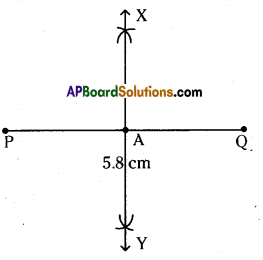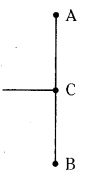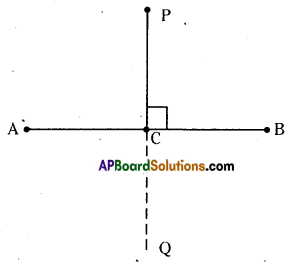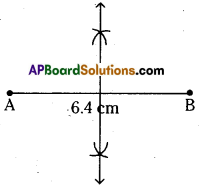AP State Syllabus AP Board 6th Class Maths Solutions Chapter 10 Practical Geometry Ex 10.2 Textbook Questions and Answers.

## AP State Syllabus 6th Class Maths Solutions 10th Lesson Practical Geometry Ex 10.2Question 1.
Draw a line segment PQ = 5.8 cm and construct its perpendicular bisector using ruler and compasses.
Solution:Steps of construction :

1. Draw a line segment $$\overline{\mathrm{PQ}}$$ = 5.8 cm.
2. Set the compasses as radius with more than half of the length of $$\overline{\mathrm{PQ}}$$.
3. With P as centre, draw arcs below and above the line segment.
4. With the same radius and Q as centre draw two arcs above and below the line segment to cut the previous arcs. Name the intersecting points of arcs as X and Y.
5. Join the points X and Y. So, the line I is the perpendicular bisector of PQ.
Hence l is required perpendicular bisector of PQ which meets at A.

Question 2.
Ravi made a line segment of length 8.6 cm. He constructed a bisector of AB on C. Find the length of AC and BC.Solution:As it is a bisector, it divides the line segment into two equal parts.
Each equal part is half of AB (8.6 cm) = $$\frac{\mathrm{AB}}{2}=\frac{8.6}{2}$$ = 4.3 cm
∴ AC = BC = 4.3 cmQuestion 3.
Using ruler and compasses, draw AB = 6.4 cm. Locate its mid point by geometric construction.
Solution:1. Draw a line segment $$\overline{\mathrm{PQ}}$$ = 6.4 cm
2. Set the compasses as radius with more than half of the length of $$\overline{\mathrm{PQ}}$$.
3. With A as centre, draw arcs below and above the line segment.
4. With the same radius and B as centre draw two arcs above and below the line segment to cut the previous arcs. Name the intersecting points of arcs as X and Y.
5. Join the points X and Y. So, the line l is the perpendicular bisector of AB.
Hence l is the required perpendicular bisector of AB which meets at M.